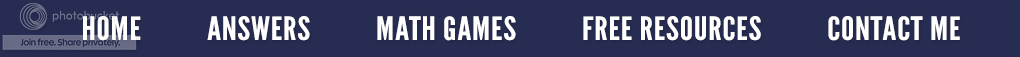### Mathematical Patterns

Since all math is based on patterns, this week, I want to target some mathematical problems in which we investigate developing patterns.

In the first example below, you will notice we begin by multiplying one by one; then 11 by 11, and so forth. Each time we multiply, the number of digits in the multiplier and the multiplicand increases. Do you see the pattern that progresses in the answer (product)? Notice how this multiplication pattern forms a triangle? Can you figure out what kind of triangle it is?

Here is another interesting pattern. In this one, instead of multiplying by 1, then 11, then 111, the answer (product) looks like the multiplier in the pattern above. Do you notice anything else significant?

Yes, we are multiplying by 9 each time. Now look at the number being added, and count the number of ones you see in each answer. Surprised? Isn’t it amazing how math is ordered, methodical and precise? Maybe that is one reason I love to teach it!